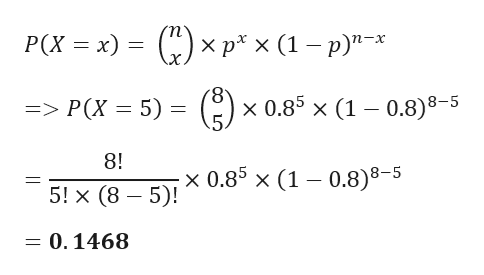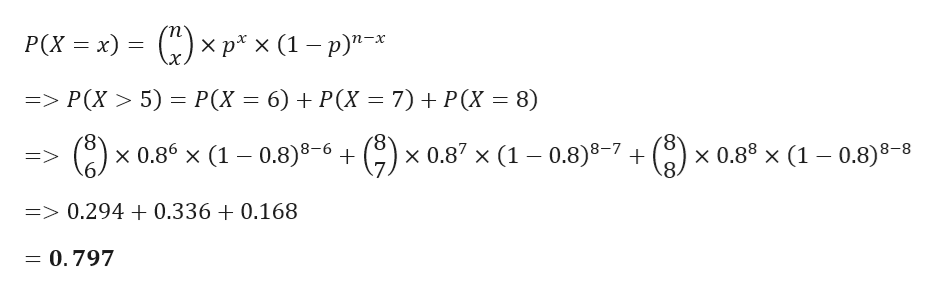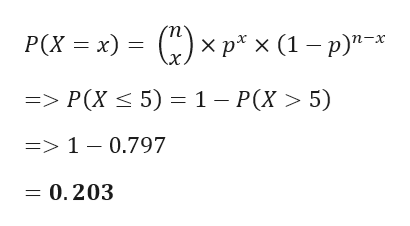# Eighty percent of households say they would feel secure if they had 50,000 in savings. You randomly select 8 households and ask them if they would feel secure if they had \$50,000 in savings. Find the probability that the number that say they would feel secure is exactly five, (b) more than five, and (c) at most fivec.) Find the probability that the number that say they would feel secure is at most five. P(<5)= (round to three decimal places as needed).

Question
15 views

Eighty percent of households say they would feel secure if they had 50,000 in savings. You randomly select 8 households and ask them if they would feel secure if they had \$50,000 in savings. Find the probability that the number that say they would feel secure is exactly five, (b) more than five, and (c) at most five

c.) Find the probability that the number that say they would feel secure is at most five. P(<5)=

(round to three decimal places as needed).

check_circle

Step 1

Given data

Probability of households feeling insecure p = 0.80

n = 8

a)

probability that the number that say they would feel secure is exactly five is given by

Applying Binomial theorem

Probability of x success out of n trial is given byhelp_outlineImage TranscriptioncloseP(X x) = ()xp* x (1 - p)"* x 0.85 x (1 0.8)8-5 => P(X 5) 8! x 0.85 x (1 - 0.8)8-5 5! x (8 5) 0.1468 fullscreen
Step 2

b)

probability that the number that say they would feel secure is more than five is given by

Applying Binomial theorem

Probability of x success out of n trial is given byhelp_outlineImage TranscriptioncloseP(X x)-()xp x (1 - p)"* х р*x (1—р)"-* => P(X> 5) = P(X = 6) + P(X 7) P(X = 8) (8 x 0.86 x (1 - 0.8)8-6 + 6 (8) x 0.87 x (1 -0.8)8-7 + x 0.88 x (1 0.8)8-8 8 => 0.294 + 0.336 + 0.168 = 0.797 fullscreen
Step 3

c)

probability that the number that say they would feel secure is at most five is given by

Applying Binomia...help_outlineImage Transcriptionclose() xp* x (1 -p)* P(X x) => P(X5) 1 - P(X > 5) => 1 - 0.797 =0.203 fullscreen

### Want to see the full answer?

See Solution

#### Want to see this answer and more?

Solutions are written by subject experts who are available 24/7. Questions are typically answered within 1 hour.*

See Solution
*Response times may vary by subject and question.
Tagged in

### Other